library: libGeom#include "TGeoTube.h"

# TGeoTube

class description - source file - inheritance tree (.pdf)

## class TGeoTube : public TGeoBBox

Inheritance Chart:
 TObject
<-
TNamed
<-
TGeoShape
<-
TGeoBBox
<-
TGeoTube
<-
 TGeoEltu
 TGeoHype
TGeoTubeSeg
<-
 TGeoCtub
```
public:
TGeoTube()
TGeoTube(Double_t rmin, Double_t rmax, Double_t dz)
TGeoTube(const char* name, Double_t rmin, Double_t rmax, Double_t dz)
TGeoTube(Double_t* params)
TGeoTube(const TGeoTube&)
virtual ~TGeoTube()
static TClass* Class()
virtual void ComputeBBox()
virtual void ComputeNormal(Double_t* point, Double_t* dir, Double_t* norm)
static void ComputeNormalS(Double_t* point, Double_t* dir, Double_t* norm, Double_t rmin, Double_t rmax, Double_t dz)
virtual Bool_t Contains(Double_t* point) const
virtual Int_t DistancetoPrimitive(Int_t px, Int_t py)
virtual Double_t DistFromInside(Double_t* point, Double_t* dir, Int_t iact = 1, Double_t step = TGeoShape::Big(), Double_t* safe = 0) const
static Double_t DistFromInsideS(Double_t* point, Double_t* dir, Double_t rmin, Double_t rmax, Double_t dz)
virtual Double_t DistFromOutside(Double_t* point, Double_t* dir, Int_t iact = 1, Double_t step = TGeoShape::Big(), Double_t* safe = 0) const
static Double_t DistFromOutsideS(Double_t* point, Double_t* dir, Double_t rmin, Double_t rmax, Double_t dz)
static void DistToTube(Double_t rsq, Double_t nsq, Double_t rdotn, Double_t radius, Double_t& b, Double_t& delta)
virtual TGeoVolume* Divide(TGeoVolume* voldiv, const char* divname, Int_t iaxis, Int_t ndiv, Double_t start, Double_t step)
virtual const char* GetAxisName(Int_t iaxis) const
virtual Double_t GetAxisRange(Int_t iaxis, Double_t& xlo, Double_t& xhi) const
virtual void GetBoundingCylinder(Double_t* param) const
virtual const TBuffer3D& GetBuffer3D(Int_t reqSections, Bool_t localFrame) const
virtual Int_t GetByteCount() const
virtual Double_t GetDz() const
virtual TGeoShape* GetMakeRuntimeShape(TGeoShape* mother, TGeoMatrix* mat) const
virtual Int_t GetNmeshVertices() const
virtual Double_t GetRmax() const
virtual Double_t GetRmin() const
Bool_t HasRmin() const
virtual void InspectShape() const
virtual TClass* IsA() const
virtual Bool_t IsCylType() const
virtual TBuffer3D* MakeBuffer3D() const
TGeoTube& operator=(const TGeoTube&)
virtual Double_t Safety(Double_t* point, Bool_t in = kTRUE) const
static Double_t SafetyS(Double_t* point, Bool_t in, Double_t rmin, Double_t rmax, Double_t dz, Int_t skipz = 0)
virtual void SavePrimitive(ofstream& out, Option_t* option)
virtual void SetDimensions(Double_t* param)
virtual void SetPoints(Double_t* points) const
virtual void SetPoints(Float_t* points) const
virtual void SetSegsAndPols(TBuffer3D& buff) const
void SetTubeDimensions(Double_t rmin, Double_t rmax, Double_t dz)
virtual void ShowMembers(TMemberInspector& insp, char* parent)
virtual void Sizeof3D() const
virtual void Streamer(TBuffer& b)
void StreamerNVirtual(TBuffer& b)
```

### Data Members

```
protected:
Double_t fDz    half length
```

## Class Description

``` TGeoTube - cylindrical tube class. It takes 3 parameters :

_____________________________________________________________________________
```
/*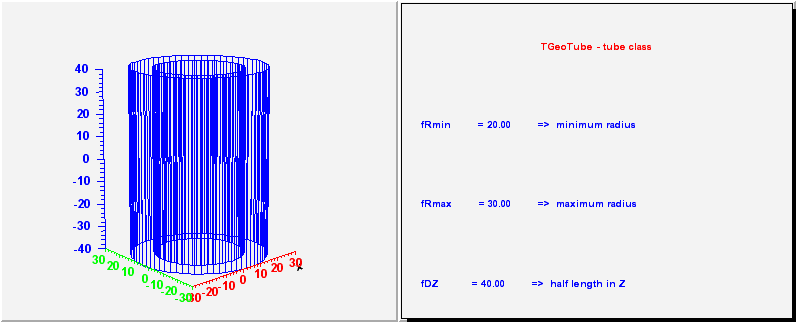*/

/*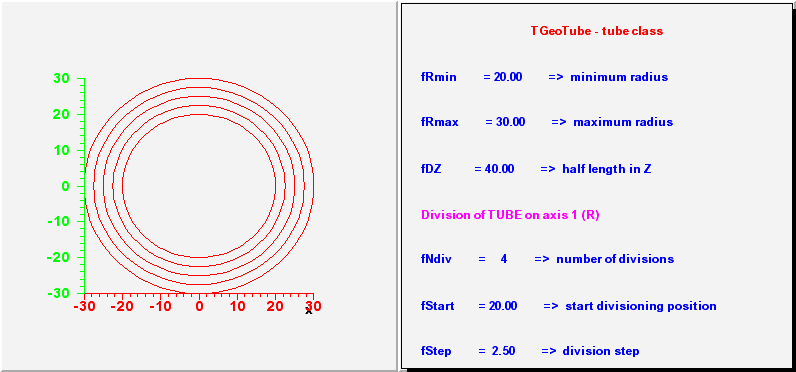*/

/*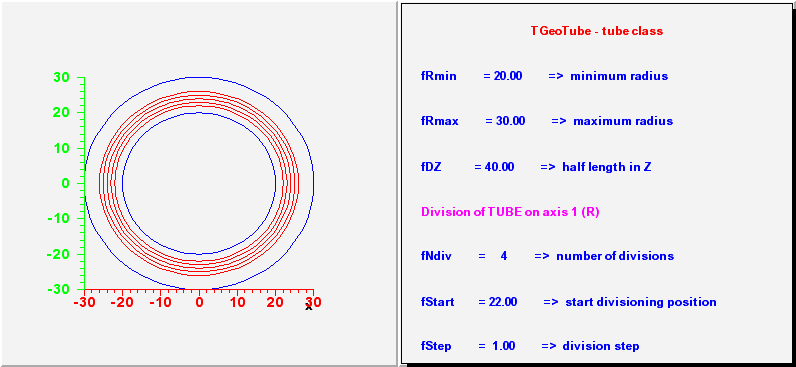*/

/*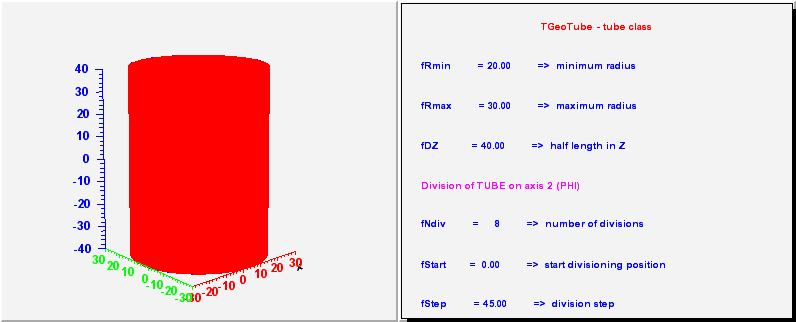*/

/*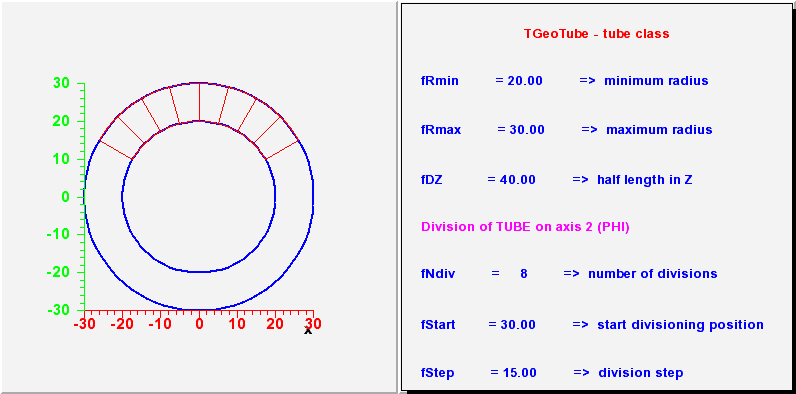*/

/*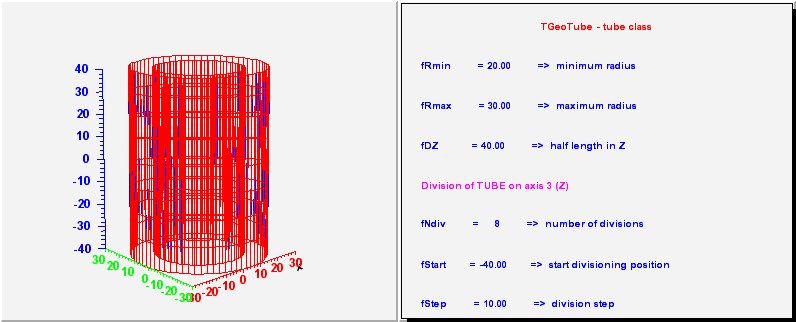*/

/**/

```_____________________________________________________________________________
TGeoTubeSeg - a phi segment of a tube. Has 5 parameters :
- the same 3 as a tube;
- first phi limit (in degrees)
- second phi limit

_____________________________________________________________________________
```
/**/

/**/

/**/

/**/

```_____________________________________________________________________________
TGeoCtub - a tube segment cut with 2 planes. Has 11 parameters :
- the same 5 as a tube segment;
- x, y, z components of the normal to the -dZ cut plane in
point (0, 0, -dZ);
- x, y, z components of the normal to the +dZ cut plane in
point (0, 0, dZ);

_____________________________________________________________________________
```
/**/

TGeoTube()
``` Default constructor
```

TGeoTube(Double_t rmin, Double_t rmax, Double_t dz) :TGeoBBox(0, 0, 0)
``` Default constructor specifying minimum and maximum radius
```

TGeoTube(const char *name, Double_t rmin, Double_t rmax, Double_t dz) :TGeoBBox(name, 0, 0, 0)
``` Default constructor specifying minimum and maximum radius
```

TGeoTube(Double_t *param) :TGeoBBox(0, 0, 0)
``` Default constructor specifying minimum and maximum radius
param = Rmin
param = Rmax
param = dz
```

~TGeoTube()
``` destructor
```

void ComputeBBox()
``` compute bounding box of the tube
```

void ComputeNormal(Double_t *point, Double_t *dir, Double_t *norm)
``` Compute normal to closest surface from POINT.
```

void ComputeNormalS(Double_t *point, Double_t *dir, Double_t *norm, Double_t /*rmin*/, Double_t /*rmax*/, Double_t /*dz*/)
``` Compute normal to closest surface from POINT.
```

Bool_t Contains(Double_t *point) const
``` test if point is inside this tube
```

Int_t DistancetoPrimitive(Int_t px, Int_t py)
``` compute closest distance from point px,py to each corner
```

Double_t DistFromInsideS(Double_t *point, Double_t *dir, Double_t rmin, Double_t rmax, Double_t dz)
``` Compute distance from inside point to surface of the tube (static)
Boundary safe algorithm.
compute distance to surface
Do Z
```

Double_t DistFromInside(Double_t *point, Double_t *dir, Int_t iact, Double_t step, Double_t *safe) const
``` Compute distance from inside point to surface of the tube
Boundary safe algorithm.
```

Double_t DistFromOutsideS(Double_t *point, Double_t *dir, Double_t rmin, Double_t rmax, Double_t dz)
``` Static method to compute distance from outside point to a tube with given parameters
Boundary safe algorithm.
check Z planes
```

Double_t DistFromOutside(Double_t *point, Double_t *dir, Int_t iact, Double_t step, Double_t *safe) const
``` Compute distance from outside point to surface of the tube and safe distance
Boundary safe algorithm.
fist localize point w.r.t tube
```

void DistToTube(Double_t rsq, Double_t nsq, Double_t rdotn, Double_t radius, Double_t &b, Double_t &delta)
``` Static method computing the distance to a tube with given radius, starting from
POINT along DIR director cosines. The distance is computed as :
RSQ   = point*point+point*point
NSQ   = dir*dir+dir*dir  ---> should NOT be 0 !!!
RDOTN = point*dir+point*dir
The distance can be computed as :
D = -B +/- DELTA
where DELTA.GT.0 and D.GT.0
```

TGeoVolume* Divide(TGeoVolume *voldiv, const char *divname, Int_t iaxis, Int_t ndiv, Double_t start, Double_t step)
```--- Divide this tube shape belonging to volume "voldiv" into ndiv volumes
called divname, from start position with the given step. Returns pointer
to created division cell volume in case of Z divisions. For radial division
creates all volumes with different shapes and returns pointer to volume that
was divided. In case a wrong division axis is supplied, returns pointer to
volume that was divided.
```

const char* GetAxisName(Int_t iaxis) const
``` Returns name of axis IAXIS.
```

Double_t GetAxisRange(Int_t iaxis, Double_t &xlo, Double_t &xhi) const
``` Get range of shape for a given axis.
```

void GetBoundingCylinder(Double_t *param) const
```--- Fill vector param with the bounding cylinder parameters. The order
is the following : Rmin, Rmax, Phi1, Phi2, dZ
```

TGeoShape* GetMakeRuntimeShape(TGeoShape *mother, TGeoMatrix * /*mat*/) const
``` in case shape has some negative parameters, these has to be computed
in order to fit the mother
```

void InspectShape() const
``` print shape parameters
```

TBuffer3D* MakeBuffer3D() const
``` Creates a TBuffer3D describing *this* shape.
Coordinates are in local reference frame.
```

void SetSegsAndPols(TBuffer3D &buffer) const
``` Fill TBuffer3D structure for segments and polygons.
```

Double_t Safety(Double_t *point, Bool_t in) const
``` computes the closest distance from given point to this shape, according
to option. The matching point on the shape is stored in spoint.
```

Double_t SafetyS(Double_t *point, Bool_t in, Double_t rmin, Double_t rmax, Double_t dz, Int_t skipz)
``` computes the closest distance from given point to this shape, according
to option. The matching point on the shape is stored in spoint.
```

void SavePrimitive(ofstream &out, Option_t * /*option*/)
``` Save a primitive as a C++ statement(s) on output stream "out".
```

void SetTubeDimensions(Double_t rmin, Double_t rmax, Double_t dz)

void SetDimensions(Double_t *param)

void SetPoints(Double_t *points) const
``` create tube mesh points
```

void SetPoints(Float_t *points) const
``` create tube mesh points
```

Int_t GetNmeshVertices() const
``` Return number of vertices of the mesh representation
```

void Sizeof3D() const
```/// fill size of this 3-D object
/    TVirtualGeoPainter *painter = gGeoManager->GetGeomPainter();
/    if (!painter) return;
/    Int_t n = gGeoManager->GetNsegments();
/    Int_t numPoints = n*4;
/    Int_t numSegs   = n*8;
/    Int_t numPolys  = n*4;
```

const TBuffer3D& GetBuffer3D(Int_t reqSections, Bool_t localFrame) const

### Inline Functions

```              Int_t GetByteCount() const
Double_t GetRmin() const
Double_t GetRmax() const
Double_t GetDz() const
Bool_t HasRmin() const
Bool_t IsCylType() const
TClass* Class()
TClass* IsA() const
void ShowMembers(TMemberInspector& insp, char* parent)
void Streamer(TBuffer& b)
void StreamerNVirtual(TBuffer& b)
TGeoTube TGeoTube(const TGeoTube&)
TGeoTube& operator=(const TGeoTube&)
```

Author: Andrei Gheata 24/10/01
Last update: root/geom:\$Name: \$:\$Id: TGeoTube.cxx,v 1.64 2005/08/30 09:58:41 brun Exp \$